# Excel SUMIF and SUMIFS – Explained

As the name suggests SUMIF and SUMIFS formulae are formed by combining SUM and IF functions. In simple English, this implies that these functions can add items or cells that fulfill a particular criterion. Let’s understand it in detail.

## Understanding SUMIF and SUMIFS Functions

You know SUM function has the capability to add items, and SUMIF function extends the capabilities of SUM function by giving you the choice to SUM only those items that meet a particular criterion.

At the same time, the SUMIFS function is a relatively newer function (introduced with Excel 2007) that extends the abilities of a SUMIF function by giving you the facility to add only those items that meet a set of criteria.

## SUMIF Function in Excel

According to Microsoft Excel SUMIF is defined as a function that “Adds the cells specified by a given condition or criteria”.

The Syntax of SUMIF Function is as under:

`=SUMIF(range, criteria [, sum_range])`

Here, ‘`range`’ refers to the cells that you want to be evaluated by the ‘`criteria`’.

`criteria`’ refers to the condition that specifies which items are to be added. ‘`criteria`’ can be a number, expression, or a text string.

`sum_range`’ is an optional argument, it specifies the cells to be added. If ‘`sum_range`’ argument is omitted then SUMIF treats ‘`range`’ as ‘`sum_range`’.

### How to use SUMIF in Excel

Now let’s try and understand how to apply a SUMIF formula.

Suppose we have a sales table as shown below:Objective: Our objective is to find the Total Sales Amount earned from the North region.

Let’s try to apply a SUMIF to find the solution.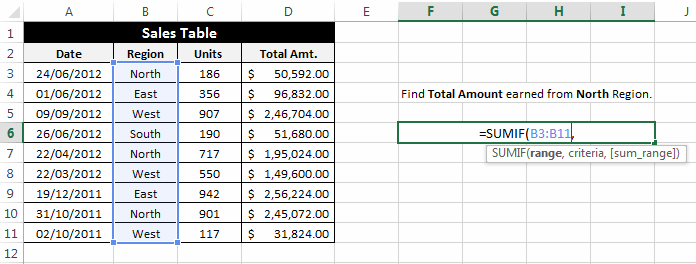range: In the ‘`range`’ argument select the cells that include your criteria.criteria: In the ‘`criteria`’ argument enter your criteria i.e. “North” Region. Please note that writing “=North” is the same as writing “North”.sum_range: In the ‘`sum_range`’ argument select the cells that should be added after evaluating the criteria.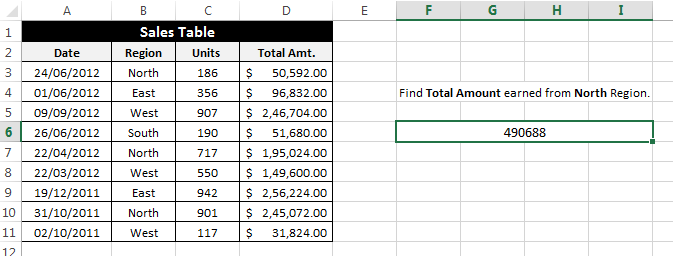After applying this formula the result comes out as 490688 which is the addition of D3, D7, and D10.

### Few Examples of SUMIF

Let’s have a look at some of the examples where SUMIF Function can be used:

Example 1: Suppose we have a table as below and now our goal is to find the total units sold in the East Region.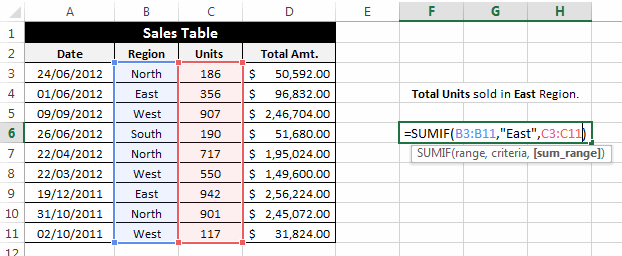To find the solution we can apply the formula as:

`=SUMIF(B3:B11,"East",C3:C11)`

This formula results into 129

Example 2: In the same table as above, write a formula to find the total amount earned before the date “01/01/2012”.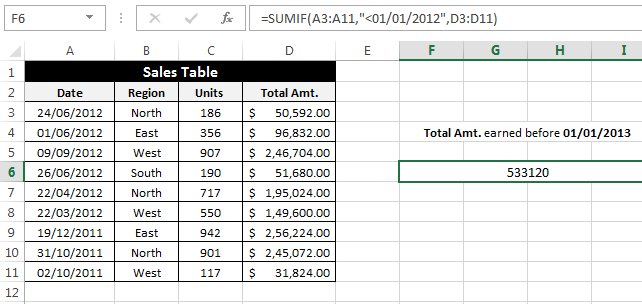In this case, we can apply the formula as:

`=SUMIF(A3:A11,"<01/01/2012",D3:D11)`

Example 3: In this example, we have a table of performance of some schools at local events. Here our task is to find the total number of awards won by School C in all the events.Here we can use a formula:

`=SUMIF(C3:C11,"School C",D3:D11)`

This formula results in 24 which is D5 + D7 + D9.

## SUMIFS Function in Excel

In the above examples we have seen how to use the SUMIF function, now let’s move to the SUMIFS function. As I have foretold that SUMIFS is an extension of SUMIF function, it has the ability to add the items from a range that satisfies multiple conditions.

According to Microsoft Excel SUMIFS can be defined as a function that “Add the cells specified by a given set of conditions or criteria”.

The syntax of the SUMIFS function is as follows:

`=SUMIFS(sum_range, criteria_range1, criteria1, [criteria_range2, criteria2], …)`

Here, ‘`sum_range`’ specifies the cells that are to be added based on the given conditions. It is a required field.

`criteria_range1`’ specifies the first range where the first criteria is to be evaluated.

`criteria1`’ specifies the condition that is to be evaluated in the ‘`criteria_range1`’.

`criteria_range2`’, ‘`criteria2`’ specifies the other ranges and their respective conditions. It is an optional argument. SUMIFS supports a total of 127 range criteria pairs.

### How to use SUMIFS in Excel

To understand how to use the SUMIFS function let’s take an example:

Let’s consider we have a Sales Table as shown below.Objective: Our objective is to find the total units sold from the North region after the date of 31/12/2011.

So, we will try to apply the SUMIFS function as:‘sum_range’: In the ‘`sum_range`’ argument select the range whose items you want to add up.‘criteria_range1’: In the ‘`criteria_range1`’ argument select the range where the first condition should be evaluated. In this case, our first condition is based on the region.‘criteria1’: In the ‘`criteria1`’ argument enter the condition that you wish to evaluate in ‘`criteria_range1`’. In our case the ‘`criteria_1`’ is “North”.‘criteria_range2’: In the ‘`criteria_range2`’ argument select the range where the second condition should be evaluated. In this example, our second condition would be based on the date.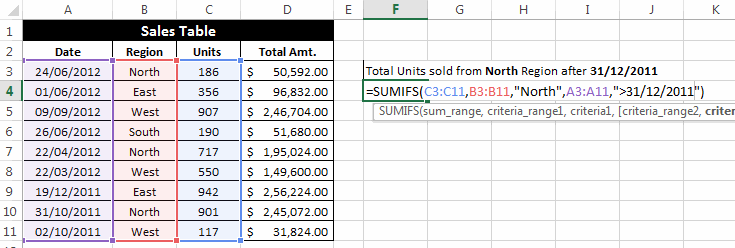‘criteria2’: In the ‘`criteria2`’ argument enter the condition that you wish to evaluate in ‘`criteria_range2`’. In our case the ‘`criteria_2`’ is “>31/12/2011”.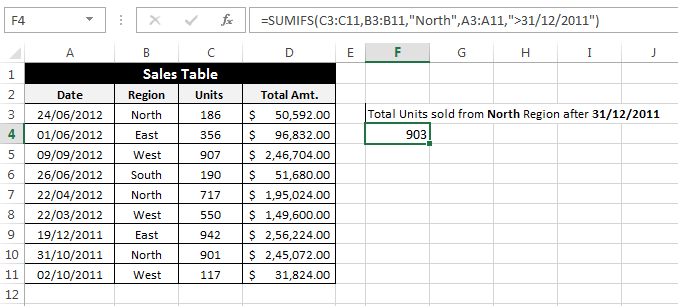The output of this formula is 903, which is the sum of C1 and C7.

### Few Examples of SUMIFS

Example 1: In this example, we again have a sales table, and our task is to find the total units sold by the salesperson “Steve” in the year 2012.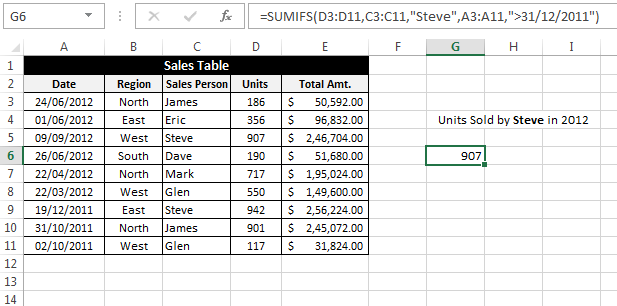We can apply a SUMIFS formula in this case as:

`=SUMIFS(D3:D11,C3:C11,"Steve",A3:A11,">31/12/2011")`

This formula gives the result of 907.

Example 2: In this example, we will try to find the total amount raised from the “West” region where items sold are greater than 500.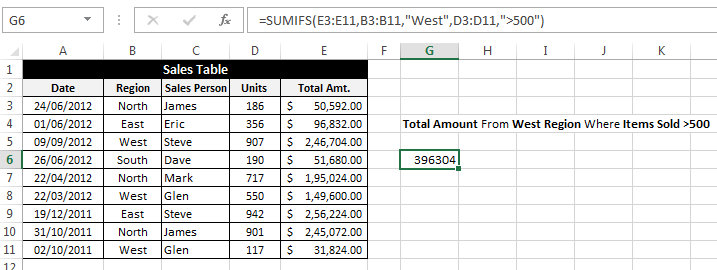In this case, we can use the formula:

`=SUMIFS(E3:E11,B3:B11,"West",D3:D11,">500")`

Example 3: In this example, we have a daily measurements table as shown below. Here our task is to find the total Rainfall for all the days where humidity is greater than 65 and temperature is greater than 40.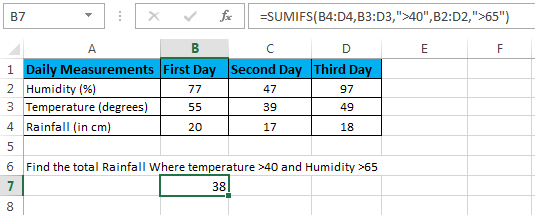To find the solution we can apply the formula:

`=SUMIFS(B4:D4,B3:D3,">40",B2:D2,">65")`

This gives 38 as the result which is a sum of B4 and D4.

## Few Important points about SUMIF and SUMIFS Formulas

• Both SUMIF and SUMIFS formulae support wildcard characters. You can use wildcard characters (like: ‘*’ and ‘?’) in the ‘`criteria`’ argument.
• In SUMIF the cells in ‘`range`’ argument and ‘`sum_range`’ need not be of the same shape and size. But this does not stand true in the case of SUMIFS.
• You can use multiple operators (like: “=”, “>”, “<”, “>=”, “<=”, “<>”) in the ‘`criteria`’ argument of both the functions.

So, this was all about Excel SUMIF and SUMIFS formulae. Feel free to drop your comments regarding the topic.About Ankit Kaul

Ankit is the founder of Excel Trick. He is tech Geek who loves to sit in front of his square headed girlfriend (his PC) all day long. :D. Ankit has a strong passion for learning Microsoft Excel. His only aim is to turn you guys into 'Excel Geeks'.

1.Rahim Zulfiqar Alisays

Thanks Ankit for this very useful lessons on Excel. I suggest that If you can also add XLSX file to download the examples mentioned above and also post some challenges so that it would be more practical and handy to learn more. Thank You

•Ankit Kaulsays

Thanks for the suggestion Rahim, I will look forward to it. 🙂

2.Rudra sharmasays

I always struggled to understand sumifs syntax.But now it is clear. Thank you…

•Ankit Kaulsays